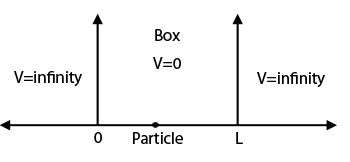## Introduction

Unlike classical (Newtonian) mechanics where there seem to be a very intuitive and wildly accepted approach of learning, quantum mechanics can be introduced through various proxies and people can learn it as either:

• Tricky model inspired by Black body radiation + Sketchy assumption
• or, Elegant (but hard to solve) equations that follow axioms of quantum mechanics.

In this 2-parts note, I will write myself a note that is most clear and logical for what I have learned so far.

For this reason, I will introduce equations and relevant mathematical tools as granted, of course with reference for them.

## Interpretation of Wave Equation

If I were to use one sentence to summarize Schrödinger equation (call it [SE] form now on):
Complex number wave function $\Psi (x,t)$ tells your probability amplitude about the particle.

This is called statistical interpretation, it does not always work, but for elementary usage of [SE], we almost can always have this image in back of our head.

Now, $\Psi$ is not straight probability because it's a complex function, to get probability you will need to give it its own conjugate, naively:
$$P(x,t) = \Psi^*\times\Psi$$

As for the reason why it has to be a complex-number function in the first place, is because if your think about $e^{i\theta} = \cos{\theta}+i\sin{\theta}$, you will see you some what need a complex function to fully express a wave function across space and time.

## Schrödinger equation and its solution

Knowing how we should look at $\Psi(x,t)$ (which is just a 1-D case but it's okay), we can look at 1-D [SE] which the wave equation $\Psi$ promised to satisfy:
$$-\frac{\hbar^2}{2m}\frac{\partial^2\Psi(x,t)}{\partial x^2} + V(x,t)\Psi = i\hbar \frac{\partial\Psi(x,t)}{\partial t}$$
Here, $\hbar$ is reduced Plank's constant, $m$ is mass of the particle and $V$ is the potential. Also bear in mind that it's a complex number equation.

We tend to think free particle solution is the simplest solution to look at but turns out that has a vague physical picture, so we look at particle in a box solution first.

But above all that, we need to invoke a mathematical (proof) that the solution is separable, meaning that $\Psi(x,t) = \psi(x)\varphi(t)$. You see physicists make this separable argument a lot, this is because they will later rescue it by proving that all such solutions combined can provide a complete solution set.

Given separable, we immediately can re-range the [SE] to get:
$$i\hbar \frac{1}{\varphi(t)} \frac{d\varphi}{dt} = -\frac{\hbar^2}{2m}\frac{1}{\psi(x)}\frac{d^2\psi(x)}{dx^2} +V$$
This suggest that left and right both equal to a constant because they are independent in variables, we will suggestively give this constant $E$. So far we have turned a two-variable ODE into 2 one-v ODEs:
$$\begin{cases} \frac{d\varphi}{dt} = \frac{iE}{\hbar}\varphi \rightarrow \varphi(t) = e^{-iEt/\hbar}\\ -\frac{\hbar^2}{2m}\frac{d^2\psi}{dx^2} +V\psi = E\psi \rightarrow ? \end{cases}$$
It turns out the second one is hard to solve (easy once you know the answer of course, it's all about tricks). The take way is, once we solved it, we will get:
$$\Psi(x,t) = \sum_{n=1}^{\infty} c_n\Psi_n(x,t) = \sum_{n=1}^{\infty} c_n\psi_n(t)e^{-iE_nt/\hbar}$$
Where you can see that the time term is snaped onto the spacial term. And all of different allowed $E_n$ will give any valid general solution.

## In a box solution

Now we consider particle in side a region that is walled by infinite potential outside:This has the convineance that potential $V=0$ inside, and we must have $\Psi=0$ out side the box (because otherwise energy explodes). Another way to look at it is that, if we know there's a particle somewhere and we accept the said interpretation, we will expect:
$$\int_{-\infty}^{\infty}|\Psi|^2 dx = \int_{-\infty}^{\infty}\Psi^*\times\Psi dx =1$$

Because there is 1 and only 1 particle in our set-up. Plus the fact that $\Psi$ should be continuous otherwise we will have different probability of spotting the particle approaching from left or right, which is un-physical. Given these 2 conditions, easy to see that out side the box, probability of finding particle must be zero and also $\psi(x=0)=\psi(x=L)=0$. This is boundry condition (b.c.)

We can focus on solving spatial part $\psi(x)$ because we can snap the time term easily later. The spacial solution is just some wave form, we look at:
$$-\frac{\hbar^2}{2m}\frac{d^2\psi}{dx^2} = E\psi$$
and write $k=\frac{\sqrt{2mE}}{\hbar}$ so $\frac{d^2\psi}{dx^2} = -k^2\psi$.

This is obviously a ODE with solution:
$$\psi = A\sin{kx} + B\cos{kx}$$
Up bring b.c. into it, we see that $B$ must goes to 0. Then because at $x=L$:
$$A\sin{k L} = 0$$
we have $k_n=\frac{n\pi}{L}$ which are discrete allowed values. From these allowed $k$'s, we can see that energy is also discrete because:
$$k=\frac{\sqrt{2mE}}{\hbar} \rightarrow E_n=\frac{\hbar^2k_n^2}{2m}$$
Then we have a whole set of solutions with different energy and wave-form, the general solution will be any combination of them:
$$\Psi(x,t) = \sum \Psi_n(x,t) = \sum \psi_n(x)\varphi_n(t) = A\sin{k_n x}e^{-iE_nt/\hbar}$$
$A$ will need to be found by normalizing this, but it shouldn't be too hard. Finally, to visualize what these different solutions mean:Notice that energy goes higher, and the form of the wave function itself actually looks like standing wave on string. But remember that probability comes from $|\Psi|^2$ not this wavefunction.